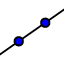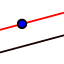# Basic Geometric Postulates

Author:
Ryan Routh
Topic:
Geometry
In this lesson, you will use Geogebra to explore some basic geometric postulates. When you get to a question that has the word "POSTULATE" in it, after checking your answer WRITE DOWN the correct formulation of that Postulate in your notes!

## POSTULATE #1: POINT-LINE POSTULATE

Look at the figure below and consider this question: Is it possible to identify a line using only one point? If I talked about "line E" in the figure below, what line would I be talking about? Click on one of the points and drag it to see if that changes your mind.

## Click and drag on a point.

If you drew as many lines as possible that pass through point E, how many lines could you draw?

Select all that apply
• A
• B
• C
• D

To name a line using points I should use...

Select all that apply
• A
• B
• C
Look at the figure below and consider these questions: How many different lines could I draw that go through points A and B? How many different lines could I draw that go through points A, B and C? (remember that "lines" in geometry means STRAIGHT lines). Try clicking on the line toolwithin the line menuand then click on various points. You can undo your work with the UNDO icon in the upper right. Now click back on the MOVE tooland drag point C around. Can you drag it to a location where a single line could be drawn that contains points A, B and C?

## Postulate #1: The Point-Line Postulate

Which of the following best describes the Point-Line Postulate?

Select all that apply
• A
• B
• C
• D

## Postulate #2: The Line Intersection Postulate

For this postulate we will consider two lines. Drag the various points around and consider this question: What are the possible number of points of intersection for two lines?

## Drag the points as instructed above.

Can two lines intersect in no points?

Select all that apply
• A
• B

Can two lines intersect at 1 point?

Select all that apply
• A
• B

Can two lines intersect at 2 points?

Select all that apply
• A
• B

Can two lines intersect at an infinite number of points?

Select all that apply
• A
• B

## Postulate #2: The Line Intersection Postulate

Which of the following best describes the postulate.

Select all that apply
• A
• B
• C

## Postulate #3: The Parallel Construction Postulate

Consider the following as you examine the applet below. What if I wanted to draw a new line that contains point C and is parallel to the line shown below? How many different new lines could I draw that satisfy these conditions?

## Postulate #3: Parallel Construction Postulate

Which of the following is true?

Select all that apply
• A
• B
• C

## Constructing parallel lines.

You can construct a parallel line automatically using Geogebra. In the toolbar of the Geogebra applet, click on the fourth box from the left and select this(the Parallel line tool). Then click on the line (in the middle away from the points) and then click on Point C.